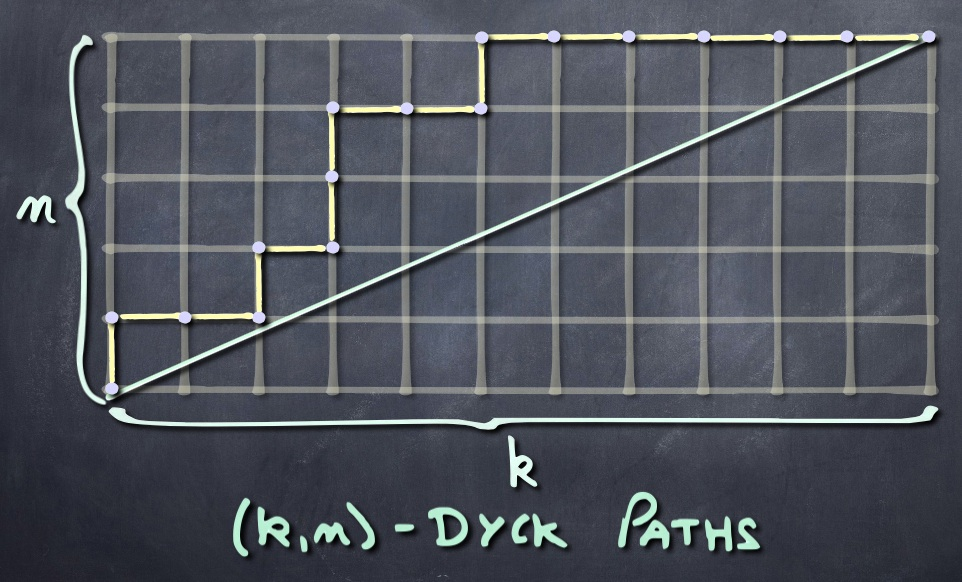# Shuffle ConjecturesThe original Shuffle Conjecture (of HHLRU, 2003) is about an explicit combinatorial description of the bigraded Frobenius characteristic $\mathcal{D}_n^m(\mathbf{x};q,t)$ of the $\mathbb{S}_n$-module of (higher) diagonal harmonic polynomials. It is stated in terms of parking functions on $(mn,n)$-Dyck paths, and involves two statistics on these: the area and the dinv. Here, $(k,n)$-Dyck paths are north-east paths in the $(k\times n)$-rectangle, going from $(0,0)$ to $(k,n)$, while remaining above the diagonal $y=n x/k$. We allow the path to return to this diagonal, but this is only possible when $k$ and $n$ are not coprime.For a given $(k,n)$-Dyck path $\gamma$, a parking function $\pi$ of that shape is simply a bijective labelling of the $n$ vertical steps of $\gamma$ by the numbers $\{1,2,\ldots,n\}$. We denote this by $\lambda(\gamma)=\pi$.

The area $\mathrm{area}(\pi)$ is the number of complete squares that lie between the path and the diagonal. The dinv-statistic is a bit more intricate (see here), and there is a composition $\mathrm{co}(\pi)$ of $n$, associated to $\pi$.  We refer to Haglund for descriptions of these, in the case $k=mn$. The most general case is in the same spirit. The final ingredient in the formula is to use Schur functions indexed by compositions. These are simply obtained using a composition analog of the Jacobi-Trudi formula:

$S_\alpha(\mathbf{x}):=\mathrm{det}\ \left(h_{\alpha_i+j-i}(\mathbf{x})\right)_{i,j}.$

One may easily check that this evaluates to either $0$ or $\pm S_\lambda(\mathbf{x})$, for some partition $\lambda$. As it has become customary, we use Macdonald notation for symmetric functions. Thus $h_j(\mathbf{x})$ stands for the complete homogenous symmetric function.

Shuffle Conjecture (HHLRU, 2003)

For all $m$ and $n$,

$\displaystyle\mathcal{D}_n^m(\mathbf{x};q,t)= \sum_{\pi=\lambda(\gamma)}t^{\mathrm{area}(\pi)}q^{\mathrm{dinv}(\pi)}\,S_{\mathrm{co}(\pi)}(\mathbf{x}),$

with $\gamma$ running through all $(mn,n)$-Dyck paths.

N. Bergeron (the other one), Descouens and Zabrocki (2010) showed that the Frobenius characteristic of the space of diagonal harmonic polynomials has a natural filtration given by the number of returns to the diagonal of Dyck paths. This takes the form of $\nabla$-operator applied to a certain symmetric function. Inspired by this, Haglund, Morse and Zabrocki proposed a refinement of the shuffle conjecture (the HMZ conjecture), involving operators $C_\alpha=C_{a_1}C_{a_2}\cdots C_{a_\ell},$ on symmetric functions, where the $C_j$ are modified Hall-Littlewood vertex operators, and $\alpha=(a_1,a_2,\ldots,a_\ell)$ is a composition of $n$. It takes the form

HMZ Conjecture (2012)

For all composition $\alpha$ of $n$,

$\displaystyle\nabla^m\ C_\alpha\cdot 1=\sum_{\mathrm{ret}(\pi)=\alpha}t^{\mathrm{area}(\pi)}q^{\mathrm{dinv}(\pi)}\,S_{\mathrm{co}(\pi)}(\mathbf{x}),$

where $\mathrm{ret}(\pi)$ is the composition that indicates returns to the diagonal in the path underlying $\pi$. This path is assumed to be a $(mn,n)$-Dyck.

In the left hand side, $C_\alpha\cdot 1$ means that the operator is applied to symmetric function $1$. This implies the Shuffle Conjecture, since it may be shown that $\sum_\alpha C_\alpha\cdot 1=e_n$, and it has been shown that $\nabla^m(e_n)=\mathcal{D}_n^m$.

Recently, using the work of Burban and Schiffmann, Gorsky and Negut have come up with an analogous conjecture involving some operators $P_{k,n}$ on symmetric functions which generalize operators first considered in our paper (with A. M. Garsia, M. Haiman, and G. Tesler):
Identities and Positivity Conjectures for some remarkable Operators in the Theory of Symmetric Functions, Methods and Applications of Analysis, 6 no. 3 (1999), 363-420. See Theorem 4.4, where the operator $\Gamma_n$ corresponds to the operator $P_{n-1,n}$ in Gorsky-Negut notation.

Rational Shuffle Conjecture (2013)

Whenever $k$ and $n$ are coprime, then

$\displaystyle P_{k,n}\cdot 1=\sum_{\pi=\lambda(\gamma)}t^{\mathrm{area}(\pi)}q^{\mathrm{dinv}(\pi)}\,S_{\mathrm{co}(\pi)}(\mathbf{x}),$

where $\gamma$ runs over $(k,n)$-Dyck paths.

Although the operators $P_{k,n}$ make sense irrespective of the coprimality condition, they are not the right ones (nor are related operators considered by Gorsky-Negut) for this last conjecture to be generalized. Together with Adriano Garsia and Emily Leven, we have come up with entirely new operators $E_{k,n},$ that do allow this conjecture to be generalized to all cases (thus removing the coprimality condition). We even have more general operators $E_{k,n}^{(\alpha)},$ for $\alpha$ any composition of $\mathrm{GCD}(k,n),$ for which we have a joint generalization of the HMZ conjecture and the Rational Shuffle Conjecture.

Compositional Rational Shuffle Conjecture (see our paper B.-Garsia-Leven-Xin)

For all integers $k$ and $n,$ and $\alpha$ composition of $\mathrm{GCD}(k,n),$

$\displaystyle E_{k,n}^{(\alpha)}\cdot 1=\sum_{\mathrm{ret}(\pi)=c\alpha}t^{\mathrm{area}(\pi)}q^{\mathrm{dinv}(\pi)}\,S_{\mathrm{co}(\pi)}(\mathbf{x}),$

where $c=n/\mathrm{GCD}(k,n),$ and $c\alpha=(ca_1,ca_2,\ldots,ca_\ell)$. The summation is over parking functions whose underlying path returns at the diagonal at the position specified by $c\alpha$.

The fact that all previous conjecture are implied by this one, follows from the operator identity $E_{k,n}=\sum_{\alpha}E_{k,n}^{(\alpha)},$ and the fact that $E_{k,n}=P_{k,n}$ when $k$ and $n$ are coprime (and only then). Furthermore, we have

$E_{k+n,n}^{(\alpha)}=\nabla\, E_{k,n}^{(\alpha)}\,\nabla^{-1},$

and $E_{0,n}^{(\alpha)}\cdot 1=C_\alpha\cdot 1$.

For more on this, see